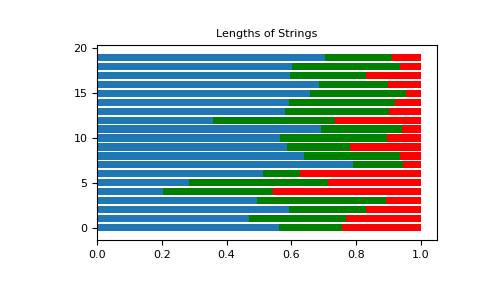#### Previous topic

numpy.random.choice

#### Next topic

numpy.random.exponential

# numpy.random.dirichlet¶

numpy.random.dirichlet(alpha, size=None)

Draw samples from the Dirichlet distribution.

Draw size samples of dimension k from a Dirichlet distribution. A Dirichlet-distributed random variable can be seen as a multivariate generalization of a Beta distribution. The Dirichlet distribution is a conjugate prior of a multinomial distribution in Bayesian inference.

Note

New code should use the dirichlet method of a default_rng() instance instead; see random-quick-start.

Parameters
alphasequence of floats, length k

Parameter of the distribution (length k for sample of length k).

sizeint or tuple of ints, optional

Output shape. If the given shape is, e.g., (m, n), then m * n * k samples are drawn. Default is None, in which case a vector of length k is returned.

Returns
samplesndarray,

The drawn samples, of shape (size, k).

Raises
ValueError

If any value in alpha is less than or equal to zero

Generator.dirichlet

which should be used for new code.

Notes

The Dirichlet distribution is a distribution over vectorsthat fulfil the conditionsand.

The probability density functionof a Dirichlet-distributed random vectoris proportional towhereis a vector containing the positive concentration parameters.

The method uses the following property for computation: letbe a random vector which has components that follow a standard gamma distribution, thenis Dirichlet-distributed

References

1

David McKay, “Information Theory, Inference and Learning Algorithms,” chapter 23, http://www.inference.org.uk/mackay/itila/

2

Wikipedia, “Dirichlet distribution”, https://en.wikipedia.org/wiki/Dirichlet_distribution

Examples

Taking an example cited in Wikipedia, this distribution can be used if one wanted to cut strings (each of initial length 1.0) into K pieces with different lengths, where each piece had, on average, a designated average length, but allowing some variation in the relative sizes of the pieces.

>>> s = np.random.dirichlet((10, 5, 3), 20).transpose()

>>> import matplotlib.pyplot as plt
>>> plt.barh(range(20), s)
>>> plt.barh(range(20), s, left=s, color='g')
>>> plt.barh(range(20), s, left=s+s, color='r')
>>> plt.title("Lengths of Strings")